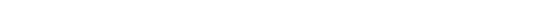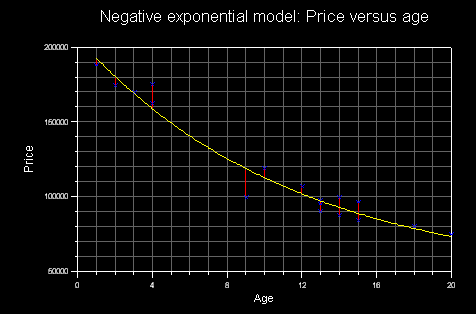NEWS NLREG has been selected as the "Editor"s Pick" by SoftSeek.NLREG is in use at hundreds of universities, laboratories, and government agencies around the world (over 20 countries). For a list of a few organizations using NLREG click here. If you have categorical variables, you may want to use a Decision Tree to model your data. Check out the DTREG Decision Tree Builder. You also should check out the News Rover program that automatically scans Usenet newsgroups, downloads messages of interest to you, decodes binary file attachments, reconstructs files split across multiple messages, and eliminates spam and duplicate files.## Introduction to Regression Analysis

The goal of regression analysis is to determine the values of parameters for a function that cause the function to best fit a set of data observations that you provide. In linear regression, the function is a linear (straight-line) equation. For example, if we assume the value of an automobile decreases by a constant amount each year after its purchase, and for each mile it is driven, the following linear function would predict its value (the dependent variable on the left side of the equal sign) as a function of the two independent variables which are age and miles:

```value = price + depage*age + depmiles*miles
```
where value, the dependent variable, is the value of the car, age is the age of the car, and miles is the number of miles that the car has been driven. The regression analysis performed by NLREG will determine the best values of the three parameters, price, the estimated value when age is 0 (i.e., when the car was new), depage, the depreciation that takes place each year, and depmiles, the depreciation for each mile driven. The values of depage and depmiles will be negative because the car loses value as age and miles increase.

For an analysis such as this car depreciation example, you must provide a data file containing the values of the dependent and independent variables for a set of observations. In this example each observation data record would contain three numbers: value, age, and miles, collected from used car ads for the same model car. The more observations you provide, the more accurate will be the estimate of the parameters. The NLREG statements to perform this regression are shown below:

```Variables value,age,miles;
Parameters price,depage,depmiles;
Function value = price + depage*age + depmiles*miles;
Data;
{data values go here}
```
Once the values of the parameters are determined by NLREG, you can use the formula to predict the value of a car based on its age and miles driven. For example, if NLREG computed a value of 16000 for price, -1000 for depage, and -0.15 for depmiles, then the function
```value = 16000 - 1000*age - 0.15*miles
```
could be used to estimate the value of a car with a known age and number of miles.

If a perfect fit existed between the function and the actual data, the actual value of each car in your data file would exactly equal the predicted value. Typically, however, this is not the case, and the difference between the actual value of the dependent variable and its predicted value for a particular observation is the error of the estimate which is known as the "deviation'' or "residual''. The goal of regression analysis is to determine the values of the parameters that minimize the sum of the squared residual values for the set of observations. This is known as a "least squares'' regression fit.

Here is a plot of a linear function fitted to a set of data values. The actual data points are marked with ''x''. The red line between a point and the fitted line represents the residual for the observation.NLREG is a very powerful regression analysis program. Using it you can perform multivariate, linear, polynomial, exponential, logistic, and general nonlinear regression. What this means is that you specify the form of the function to be fitted to the data, and the function may include nonlinear terms such as variables raised to powers and library functions such as log, exponential, sine, etc. For complex analyses, NLREG allows you to specify function models using conditional statements (if, else), looping (for, do, while), work variables, and arrays. NLREG uses a state-of-the-art regression algorithm that works as well, or better, than any you are likely to find in any other, more expensive, commercial statistical packages.

As an example of nonlinear regression, consider another depreciation problem. The value of a used airplane decreases for each year of its age. Assuming the value of a plane falls by the same amount each year, a linear function relating value to age is:

```value = p0 + p1*Age
```
Where p0 and p1 are the parameters whose values are to be determined. However, it is a well-known fact that planes (and automobiles) lose more value the first year than the second, and more the second than the third, etc. This means that a linear (straight-line) function cannot accurately model this situation. A better, nonlinear, function is:
```value = p0 + p1*exp(-p2*Age)
```
Where the ''exp'' function is the value of e (2.7182818...) raised to a power. This type of function is known as "negative exponential" and is appropriate for modeling a value whose rate of decrease is proportional to the difference between the value and some base value. Here is a plot of a negative exponential function fitted to a set of data values.Much of the convenience of NLREG comes from the fact that you can enter complicated functions using ordinary algebraic notation. Examples of functions that can be handled with NLREG include:
```Linear:        Y = p0 + p1*X
Quadratic:     Y = p0 + p1*X + p2*X^2
Multivariate:  Y = p0 + p1*X + p2*Z + p3*X*Z
Exponential:   Y = p0 + p1*exp(X)
Periodic:      Y = p0 + p1*sin(p2*X)
Misc:          Y = p0 + p1*Y + p2*exp(Y) + p3*sin(Z)
```
In other words, the function is a general expression involving one dependent variable (on the left of the equal sign), one or more independent variables, and one or more parameters whose values are to be estimated. NLREG can handle up to 500 variables and 500 parameters.

Because of its generality, NLREG can perform all of the regressions handled by ordinary linear or multivariate regression programs as well as nonlinear regression.

Some other regression programs claim to perform nonlinear regression but actually do it by transforming the values of the variables such that the function is converted to linear form. They then perform a linear regression on the transformed function. This technique has a major flaw: it determines the values of the parameters that minimize the squared residuals for the transformed, linearized function rather than the original function. This is different than minimizing the squared residuals for the actual function and the estimated values of the parameters may not produce the best fit of the original function to the data. NLREG uses a true nonlinear regression technique that minimizes the squared residuals for the actual function. Also, NLREG can handle functions that cannot be transformed to a linear form. < --> Error: #include file specification missing closing quote <-- /tbody>